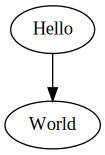# Coding Interview Tips

First things first, ask questions before jumping into coding.

• Clarify assumptions and constraints.
• Propose naive solution.

## Solve the Problem

### Use Built-in Functions

``````__builtin_popcount
__builtin_parity
__builtin_clz
__builtin_ctz``````

### Overflow

See the reverse integer problem for some basic overflow avoidance logic. And the sqrt(x) problem for more advanced overflow when you need to take into account the division and the denominator can be zero so that has to be treated separately.

### Underflow

`a.size() - b.size()` can underflow because they’re both `size_t`, so you must do `(int)(a.size() - b.size())`.

### Free Up Nodes

It’s often forgotted, but when you remove a node from a linked list, you must free up its memory. Use `delete`.

### Two's Complement

To calculate two's complement of a negative number in C++, do `(uint)num` — it's as simple as that.

### Convert Letter to Uppercase

The result of `'a' & '_' == 'A'` and even if the letter is already uppercase `'A' & '_' == 'A'`. So, it's safe to bitwise and underscore to convert a letter to its uppercase representation.

Use the `std::assert` function as replacement for a unit test framework; `std::assert` will call `std::abort` in case it fails.

Note that there’s also `std::static_assert` which is a compile-time assertion function.

``````assert(1 + 2 == 3);
``````

## Improve Runtime Performance

For the curious mind, please see the full list of options that control optimization on the GNU GCC website.

``````#pragma GCC optimize("Ofast,unroll-loops,max-inline-insns-recursive")
#pragma GCC target("avx,avx2,fma")

static const int _=[](){ios::sync_with_stdio(false);cin.tie(nullptr);cout.tie(nullptr);return 0;}();
``````

The following optimization levels are available:

 `-O1` `-O2` `-O3` `-Ofast` `-fauto-inc-dec` `-fcompare-elim` `-fcprop-registers` `-fdce` `-fdefer-pop` `-fdelayed-branch` `-fdse` `-fguess-branch-probability` `-fif-conversion2` `-fif-conversion` `-fipa-pure-const` `-fipa-profile` `-fipa-reference` `-fmerge-constants` `-fomit-frame-pointer` `-fsplit-wide-types` `-ftree-bit-ccp` `-ftree-builtin-call-dce` `-ftree-ccp` `-ftree-ch` `-ftree-copyrename` `-ftree-dce` `-ftree-dominator-opts` `-ftree-dse` `-ftree-forwprop` `-ftree-fre` `-ftree-phiprop` `-ftree-slsr` `-ftree-sra` `-ftree-pta` `-ftree-ter` `-funit-at-a-time` All in `-O1`, and: `-falign-functions` `-falign-jumps` `-falign-labels` `-falign-loops` `-fcaller-saves` `-fcrossjumping` `-fcse-follow-jumps` `-fcse-skip-blocks` `-fdelete-null-pointer-checks` `-fdevirtualize` `-fexpensive-optimizations` `-fgcse` `-fgcse-lm` `-fhoist-adjacent-loads` `-findirect-inlining` `-finline-small-functions` `-fipa-sra` `-foptimize-sibling-calls` `-fpartial-inlining` `-fpeephole2` `-fregmove` `-freorder-blocks` `-freorder-functions` `-frerun-cse-after-loop` `-fsched-interblock` `-fsched-spec` `-fschedule-insns` `-fschedule-insns2` `-fstrict-aliasing` `-fstrict-overflow` `-fthread-jumps` `-ftree-switch-conversion` `-ftree-tail-merge` `-ftree-pre` `-ftree-vrp` All in `-O2`, and: `-fgcse-after-reload` `-finline-functions` `-fipa-cp-clone` `-fpredictive-commoning` `-ftree-partial-pre` `-ftree-vectorize` `-funswitch-loops` `-fvect-cost-model` All in `-O3`, and: `-ffast-math` `-fno-protect-parens` `-fstack-arrays`
• `std::ios::sync_with_stdio` sets whether the standard C++ streams are synchronized to the standard C streams after each input/output operation. If the synchronization is turned off, the C++ standard streams are allowed to buffer their I/O independently, which may be considerably faster in some cases. By default, all eight standard C++ streams are synchronized with their respective C streams. Thus, we turn off the sync for performance gains because we don't care about printing to the standard output during interviews.
• `std::cin.tie(nullptr)` and `std::cout.tie(nullptr)` unties `std::cin` from `std::cout` so that streams aren't flushed between input/output operations.

#### Measuring Performance Improvements

``````#include <ctime>
#include <iostream>

using namespace std;

int main()
{
clock_t t0 = clock();
long result;

for (int i = 0; i < 1e6; ++i) {
for (int j = 0; j < 1e3; ++j) {
result = i * j;
}
}

clock_t t1 = clock();

cout << result << endl;
cout << "Time: " << fixed << (double)(t1 - t0) / CLOCKS_PER_SEC << " seconds" << endl;
}
``````

And here are the results:

``````g++ -O0 -Wall performance.cc -o performance.out && ./performance.out
998999001
Time: 0.487458 seconds
``````
``````g++ -O1 -Wall performance.cc -o performance.out && ./performance.out
998999001
Time: 0.230510 seconds
``````
``````g++ -O2 -Wall performance.cc -o performance.out && ./performance.out
998999001
Time: 0.000001 seconds
``````
``````g++ -O3 -Wall performance.cc -o performance.out && ./performance.out
998999001
Time: 0.000001 seconds
``````
``````g++ -Ofast -Wall performance.cc -o performance.out && ./performance.out
998999001
Time: 0.000001 seconds
``````

## Draw Diagrams

If required, and only if required, draw diagrams.